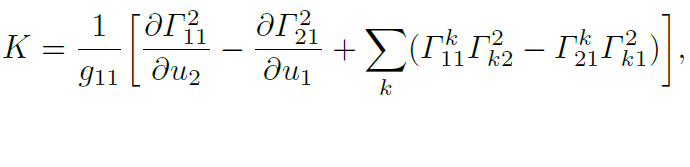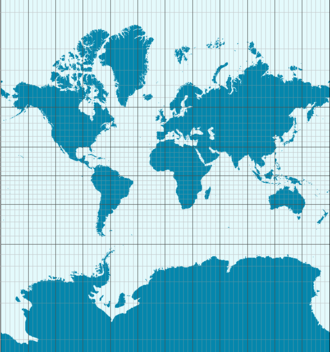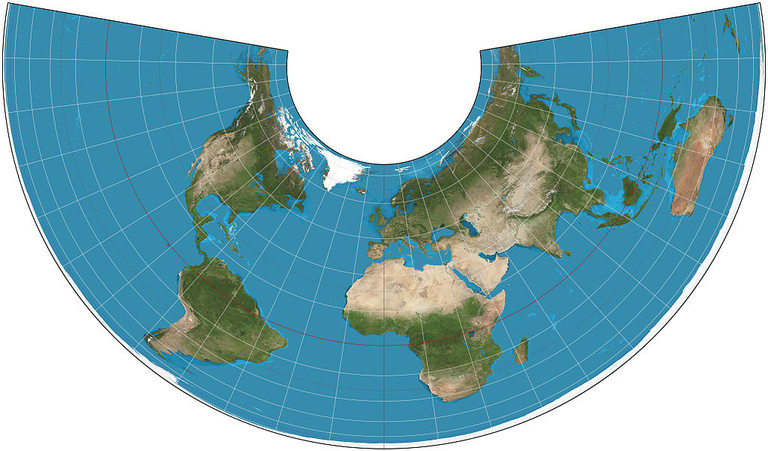# Theorema egregium - trace of advanced mathematics in real life.

in #science2 years ago (edited)

If you have an apple and I have an apple and we exchange these
apples then you and I will still each have one apple. But if you
have an idea and I have an idea and we exchange these ideas, then
each of us will have two ideas.
[G.B. Shaw]

Theorema egregium is a math theorem from 1827, made by one of best mathematicians ever - Carl Friedrich Gauss. That article will be about it, but I will write nor proof neither the ideas behind it. The reason is simple - I'm not proper person. Practically, I got interested in differential geometry just after I read about that theorem (however it was not yesterday, some things I already know).

In introduction to his book "Calculus on Manifolds", Michael Spivak wrote something like this: "You can easily spot a difference between beginner's mathematics and advanced mathematics. In beginner's mathematics the definitions are easy and theorems are hard. In advanced mathematics, the definitions are hard and theorems are easy (if You've put enough effort to understand the definitions and their consequences earlier)."

Under Spivak's words, Theorem egregium belongs to advanced mathematics, but its consequences can be seen in real life.
Here is the formulation of Theorema Egregium from the book I've been using recently:Not even one word :) Just symbols. If You understand all symbols, You understand the statement of the theorem without any additional work.

Okay, but let's go to the most interesting part.
You have probably noticed that something is not right about flat maps of the Earth. Usually, the Greenland, Siberia and Northern Canada seem to be to big, right?
Theorema egregium means that You CANNOT make a proper flat map of the Earth (which from mathematical point of view is very close to sphere, the importance is that it is has nonzero curvature, while plane has zero curvature). By the way, curvature stands for K in Theorema egregium equation).

Every map of Earth must in some place lie about angles or about distances. Or about both of them.The map above (taken from English Wikipedia) does not lie about angles but lies about distances - lands near to the poles are too vast and lands near to the equator are too small.The map above preserves distance but not angles. (also taken from English Wikipedia).

Thanks for reading that article. If You have any questions or suggestions, feel free to ask them in the comment.